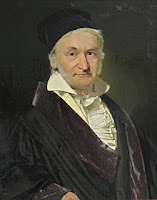## Monday, April 30, 2018

### Johann Carl Friedrich Gauß Google doodle honors mathematician known as the ‘Prince of Mathematicians’ | Search Engine Land

"The celebrated mathematician made contributions across several mathematical fields" inform Amy Gesenhues, Third Door Media's General Assignment Reporter.Photo: Search Engine Land

Today’s Google doodle marks the 241st birthday of Johann Carl Friedrich Gauß, the German mathematician often credited as the “Prince of Mathematicians” or the “Greatest mathematician since antiquity.”

Born on this date in Braunschweig, Germany, Gauß (translated as Gauss) was a child prodigy, making complex mathematical calculations as early as 8 years old. At 21, he wrote “Disquisitiones Arithmeticae,” a number theory textbook defined by Yale Press University as the “… source of ideas from which number theory was developed.”

The celebrated mathematician is noted for a number of contributions across multiple fields of study, including number theory, algebra, statistics, geometry, geophysics, magnetic fields and astronomy. Among his many discoveries was the construction of the heptadecagon and proof of the quadratic reciprocity law. According to Leonard Bruno and Lawrence Baker’s “Math and Mathematicians: The History of Math Discoveries Around the World,” Gauß determined the orbit of the asteroid Ceres in 1801.Photo: Carl Friedrich Gauß (1777–1855), painted by Christian Albrecht Jensen